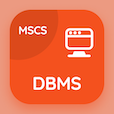Cambridge Online Courses (GCSE)

O Level Physics Quizzes

O Level Physics Quiz PDF - Complete

# Temperature Scales Quiz Questions Online p. 115

Learn Temperature Scales quiz questions and answers, temperature scales MCQ with answers PDF 115 to study O Level Physics course online. Temperature in Physics trivia questions, temperature scales Multiple Choice Questions (MCQ) for online college degrees. "Temperature Scales Quiz" PDF Book: introduction to sound, latent heat, introduction to pressure, what is temperature, temperature scales test prep for free online college courses.

"Steam point is equal to 373 K, which is equal to" MCQ PDF: 100 °c, −100 °c, 473 °c, and −473 °c for colleges that offer online degrees. Study temperature in physics questions and answers to improve problem solving skills for online colleges that offer certificate programs.

## Quiz on Temperature Scales MCQs

MCQ: Steam point is equal to 373 K, which is equal to

−100 °C
100 °C
473 °C
−473 °C

MCQ: To set up temperature of a thermometer

Melting and boiling points of water are used
Melting and boiling point of mercury
Fixed points are used
Absolute zero is used

MCQ: Pressure is defined as

area covered per unit force
force acting per unit area
distance covered per unit force
force acting per unit distance

MCQ: Unit for latent heat is

Watts
Joules
Ohms
Pascal

MCQ: Compressions and rarefactions are due to the

movement if air molecules
vibration of air molecules
variability of air pressure
variability of vibration of air molecules

### More Quizzes from O Level Physics Course